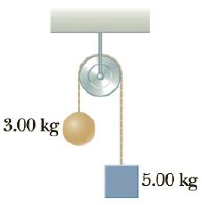Chapter 4, Problem 66P

Chapter
Section
Textbook Problem

Two objects with masses of 3.00 kg and 5.00 kg are connected by a light string that passes over a frictionless pulley, as in Figure P4.66. Determine (a) the tension in the string, (b) the acceleration of each object, and (c) the distance each object will move in the first second of motion if both objects start from rest.Figure P4.66

(a)

To determine
The tension in the string.

Explanation

Given info: The masses are m1 = 3.00 kg and m2 = 5.00 kg.

The free body diagram is given below.

From the free body diagram,

Tm1g=m1a (I)

m2gT=m2a (II)

• m1 and m2 are the masses of blocks.
• a is the acceleration.
• g is the acceleration due to gravity.
• T is the tension in the cable.

Re-arrange Equation (II) to get a.

a=g(Tm2) (III)

Substitute Equation (III) in (I) and re-arrange to get T.

T=g(2m1m2m1+m2)

Substitute 9

(b)

To determine
The acceleration.

(c)

To determine
The distance moved after one second.

Still sussing out bartleby?

Check out a sample textbook solution.

See a sample solution

The Solution to Your Study Problems

Bartleby provides explanations to thousands of textbook problems written by our experts, many with advanced degrees!

Get Started

Find more solutions based on key concepts